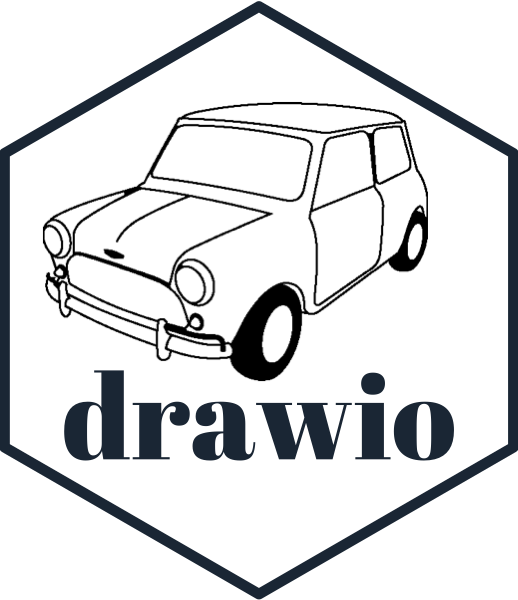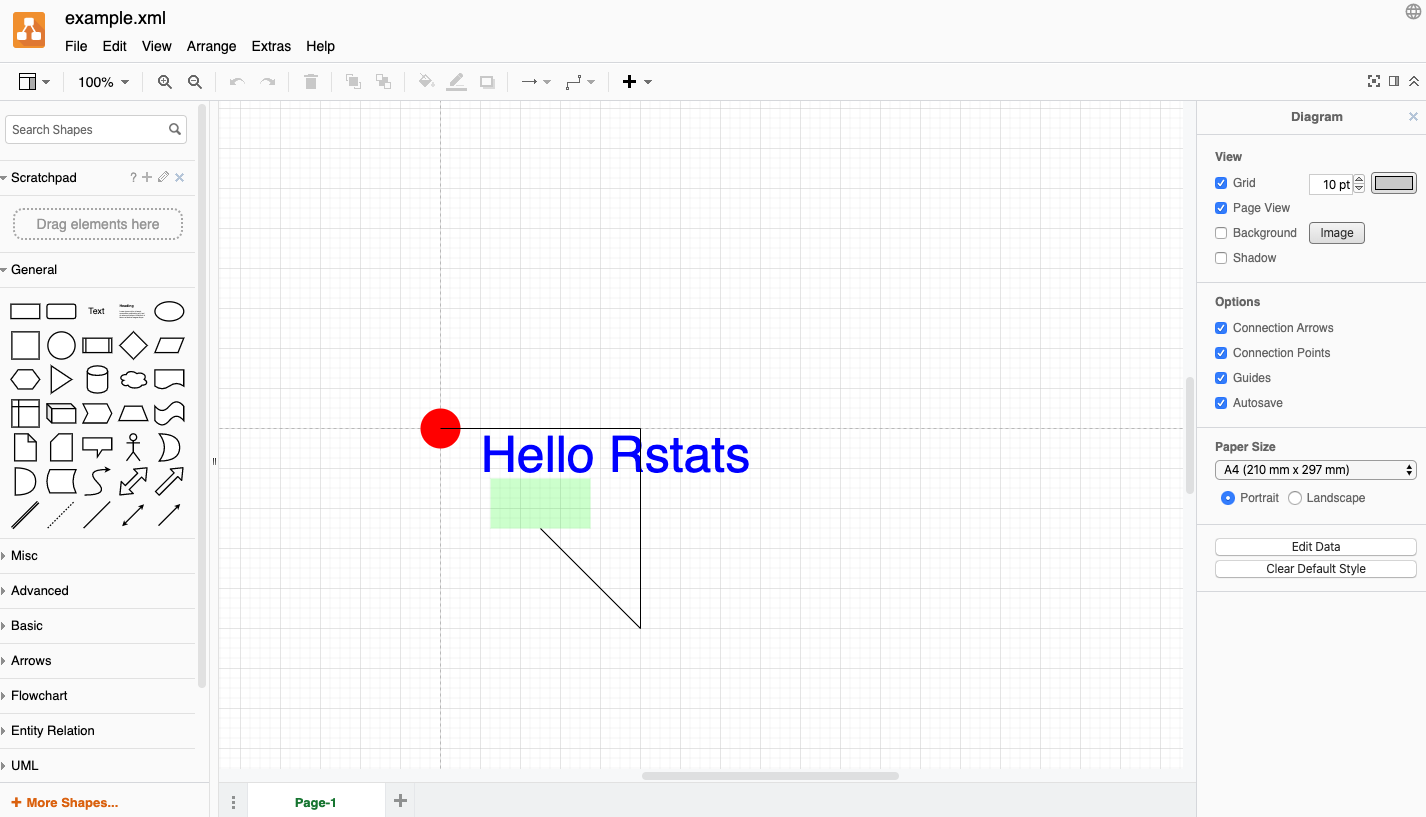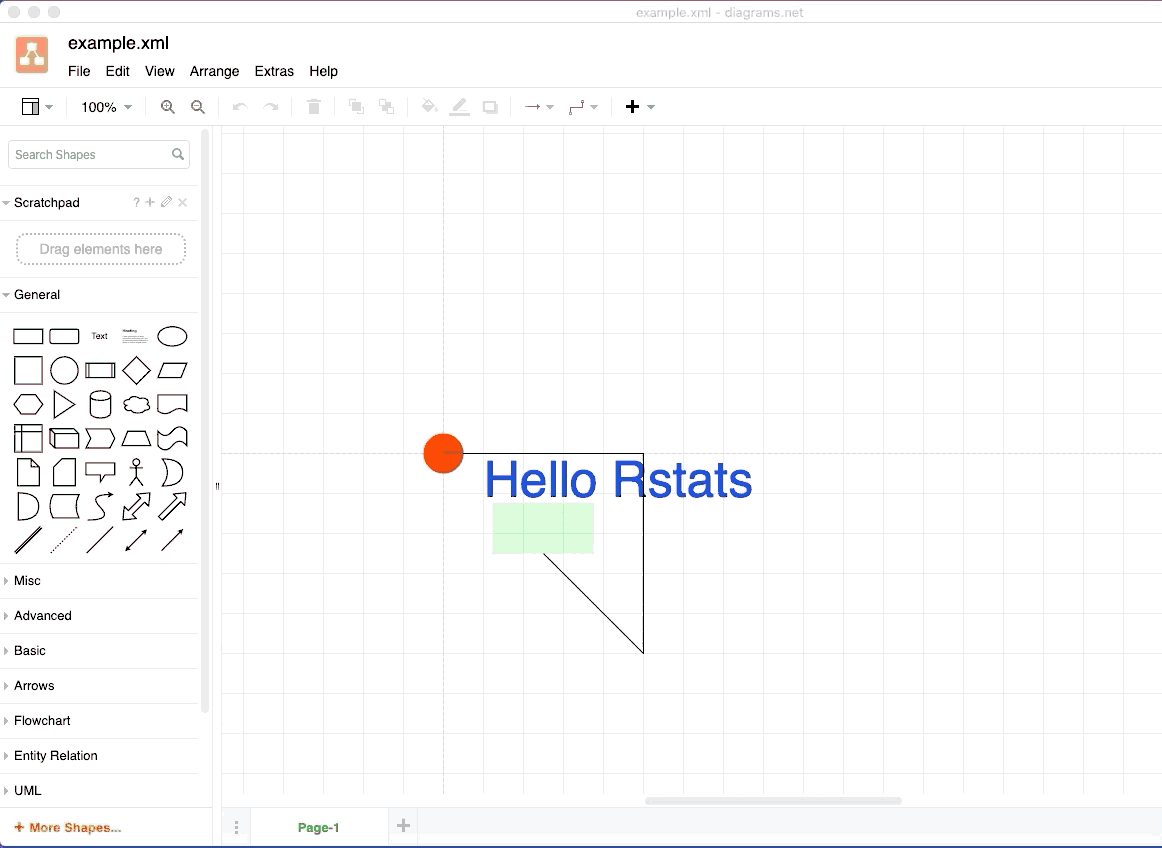# minidrawio#### minidrawio is a package for creating simple, single-page draw.io documents.

draw.io is a vector diagram format and a website for editing such diagrams.

minidrawio is an R package which allows you to create draw.io diagrams.

It’s killer feature is that the draw.io website is an interactive vector diagram editor. Any diagram you create with minidrawio can be uploaded to the site (for free!) and edited.

Currently, minidrawio is not a very complete implementation. Its initial purpose is to contain enough functionality to be able to use it as a graphics device backend. (devoutdrawio is coming soon!)

## Installation

You can install from GitHub with:

# install.packages("remotes")
remotes::install_github("coolbutuseless/minidrawio")

## Future possibilities

• Lots more documentation and examples
• Feature parity with what the draw.io web interface offers.
• e.g. be able to programatically add any of the library of shapes and link them together correctly in a hierarchy.
• text rotation, shadows, grid layouts, etc, etc.

## Simple example of building a draw.io document

#~~~~~~~~~~~~~~~~~~~~~~~~~~~~~~~~~~~~~~~~~~~~~~~~~~~~~~~~~~~~~~~~~~~~~~~~~~~~~~
# This is the basic setup needed for every drawio file
#~~~~~~~~~~~~~~~~~~~~~~~~~~~~~~~~~~~~~~~~~~~~~~~~~~~~~~~~~~~~~~~~~~~~~~~~~~~~~~
doc   <- DrawIODocument$new() dia <- doc$diagram(id = 'aaa')
graph <- dia$mxGraphModel() root <- graph$root()
root$mxCell(id = 0) root$mxCell(id = 1, parent = 0)

#~~~~~~~~~~~~~~~~~~~~~~~~~~~~~~~~~~~~~~~~~~~~~~~~~~~~~~~~~~~~~~~~~~~~~~~~~~~~~~
# Now add some text and other elements
#~~~~~~~~~~~~~~~~~~~~~~~~~~~~~~~~~~~~~~~~~~~~~~~~~~~~~~~~~~~~~~~~~~~~~~~~~~~~~~
root$rect(x = 50, y = 50, width = 100, height = 50, size = 0, colour = NA, fill = 'green', alpha = 0.2) root$text(x = 150, y = 50, colour = 'blue', text = "Hello Rstats", fontsize = 50)
root$line(x1 = 100, y1=100, x2=200, y2 = 200) root$circle(0, 0, 20, fill = 'red')
root$polygon(c(0, 200, 200), c(0, 0, 200), fill = 'none') #~~~~~~~~~~~~~~~~~~~~~~~~~~~~~~~~~~~~~~~~~~~~~~~~~~~~~~~~~~~~~~~~~~~~~~~~~~~~~~ # Save to drawio/XML format #~~~~~~~~~~~~~~~~~~~~~~~~~~~~~~~~~~~~~~~~~~~~~~~~~~~~~~~~~~~~~~~~~~~~~~~~~~~~~~ doc$save("/img/minidrawio/example.xml")

You can download the xml, or click the link below to take you directly to the plot on draw.io

Click to view/edit the plot on draw.io

#### Screenshot of plot in draw.io# Edit in drawio

Now that the diagram is saved in drawio format, we can load it up in the drawio web app and edit it to our heart’s content!